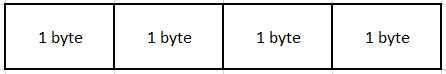In this tutorial, we are going to learn about union and how is it different from a structure.

## Union in C:

• A union is also a collection of different data types in C but that allows to store of different data types in the same memory location.
• User can define a union with many members, but only one member can contain a value at any given time.
• Unions provide an efficient way of using the same memory location for multiple-purpose.
• A union is the same as structures but the difference is that only one member can be accessed at a time because the memory is created only for one member which has the highest number of bytes in size.
• A union is declared by using the keyword union and members of the union can be accessed by using the dot (.) operator.

The declaration and definition of the union:

``````union tag_name {
data_type1 var1;
data_type2 var2;
......
data_typen varn;
};``````

Below code is an example for union:

``````union example {
int number;
float price;
char ch;
};
union example e;
``````

In the above example 4 bytes of memory is allocated to the union variable e, the members can be accessed as e.numbere.pricee.ch but only one member can be accessed at a time because the same memory is used for all the 3 members.Let us consider an example:

``````#include <stdio.h>
#include <stdlib.h>

union employee {
int age;
float sal;
};
void main() {
union employee e1;
printf("Enter age :- ");
scanf("%d", &e1.age);
printf("Age is %d\n", e1.age);
printf("Enter salary :- ");
scanf("%f", &e1.sal);
printf("Salary is %f\n", e1.sal);
}``````

Output:

``````Enter age :- 32
Age is 32
Enter salary :- 12300
Salary is 12300.000000``````

### Points to Note:

• Structure is a derived data type to organize a group of related data items of different data types referring to a single entity. i.e., a single variable capable of holding data items of different data types.
• Union is also a collection of different data types but only one member can be accessed at a time.
• An array is a collection of elements of the same data type.

In C, the user can specify the size in bits for the members of a structure and a union.

The idea behind the usage of bits is to use memory efficiently when the value of a field does not exceed the limit of storing the bits.

Bit fields can be used to reduce memory consumption when a program requires a number of integer variables which will always consist of low values.

For example, consider the following example:

``````#include <stdio.h>
struct date {
int day;
int month;
int year;
};
void main() {
struct date d = {01, 12, 2001};
printf("Given date is: %d/%d/%d\n", d.day, d.month, d.year);
}``````

Output:

``Given date is: 1/12/2001``

The size of the above structure is 12 bytes ( in a 32-bit processor) because each int type occupies 4 bytes of memory.

Here, the value of the day is always from 1 to 31 and the value of the month is from 1 to 12. hence, space can be optimized by using bit fields.

The field day will take a maximum of 5 bits to store the value between 1 and 31, as well as the month will take a maximum of 4 bits to store the value between 1 and 12. So, represent them in bits and not in bytes.

The declaration of a bit field in the structure is as follows:

``````struct tag_name {
data_type member_name : width_in_bits;
// width_in_bits specifies the number of bits in the bit-field. The width must be less than or equal to the bit width of the specified type
};``````

The above example can be written as:

``````#include <stdio.h>
struct date {
unsigned int day : 5;
unsigned int month : 4;
unsigned int year;
};
void main() {
struct date d = {01, 12, 2001};
printf("Given date is: %d/%d/%d\n", d.day, d.month, d.year);
printf("The size of the given structure %d\n", sizeof(struct date));
}``````

Output:

``````Given date is: 1/12/2001
The size of the given structure 8
``````

We cannot have pointers to bit field members as they may not start at a byte boundary.
Consider the following example:

``````struct test {
unsigned int x: 5;
unsigned int y: 5;
unsigned int z;
};
void main() {
test t;
printf("Address of t.x is %u\n", &t.x); // It results in the following error - cannot take address of bit-field x
}``````

When the value of a field exceeds the limit, it will take a garbage value and access it. Consider another example:

``````struct {
unsigned int age : 3;
} age;``````

The above structure definition instructs the C compiler that the age variable is going to use only 3 bits to store the value. If you try to use more than 3 bits, then it will use a garbage value. An array of bit fields is not allowed.
For example, the given program fails during compilation.

``````struct test {
unsigned int x : 5; //Error
};
``````

Happy Learning 🙂

### About the Author: Hemanth Krishna Vineel RamayanamI’m an Undergraduate Student at the Indian Institute of Information Technology, Design, and Manufacturing, Kurnool, Andhra Pradesh. I belong to the CSE domain.